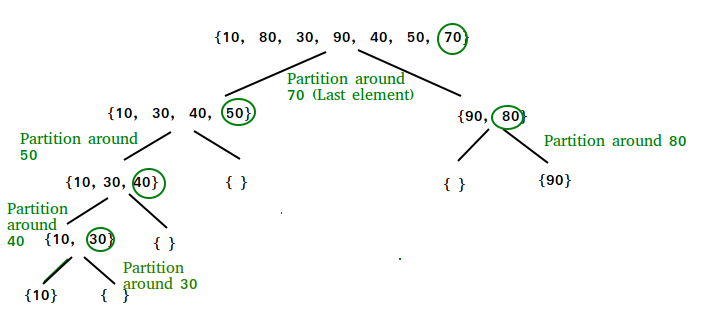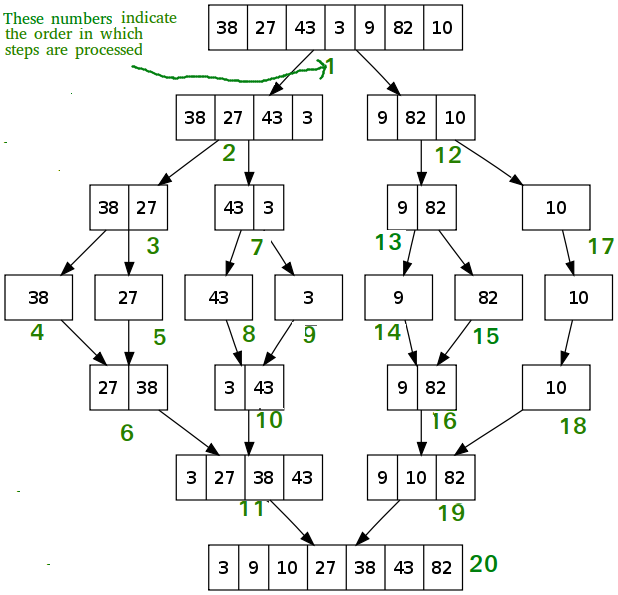# Quick Sort vs Merge Sort

• Difficulty Level : Easy
• Last Updated : 20 Jun, 2022
Prerequisite :Merge Sort and Quick Sort

Quick sort is an internal algorithm which is based on divide and conquer strategy. In this:

• The array of elements is divided into parts repeatedly until it is not possible to divide it further.
• It is also known as “partition exchange sort”.
• It uses a key element (pivot) for partitioning the elements.
• One left partition contains all those elements that are smaller than the pivot and one right partition contains all those elements which are greater than the key element.Merge sort is an external algorithm and based on divide and conquer strategy. In this:

• The elements are split into two sub-arrays (n/2) again and again until only one element is left.
• Merge sort uses additional storage for sorting the auxiliary array.
• Merge sort uses three arrays where two are used for storing each half, and the third external one is used to store the final sorted list by merging other two and each array is then sorted recursively.
• At last, the all sub arrays are merged to make it ‘n’ element size of the array.### Quick Sort vs Merge Sort

1. Partition of elements in the array :
In the merge sort, the array is parted into just 2 halves (i.e. n/2).
whereas
In case of quick sort, the array is parted into any ratio. There is no compulsion of dividing the array of elements into equal parts in quick sort.
2. Worst case complexity :
The worst case complexity of quick sort is O(n2) as there is need of lot of comparisons in the worst condition.
whereas
In merge sort, worst case and average case has same complexities O(n log n).
3. Usage with datasets :
Merge sort can work well on any type of data sets irrespective of its size (either large or small).
whereas
The quick sort cannot work well with large datasets.
4. Additional storage space requirement :
Merge sort is not in place because it requires additional memory space to store the auxiliary arrays.
whereas
The quick sort is in place as it doesn’t require any additional storage.
5. Efficiency :
Merge sort is more efficient and works faster than quick sort in case of larger array size or datasets.
whereas
Quick sort is more efficient and works faster than merge sort in case of smaller array size or datasets.
6. Sorting method :
The quick sort is internal sorting method where the data is sorted in main memory.
whereas
The merge sort is external sorting method in which the data that is to be sorted cannot be accommodated in the memory and needed auxiliary memory for sorting.
7. Stability :
Merge sort is stable as two elements with equal value appear in the same order in sorted output as they were in the input unsorted array.
whereas
Quick sort is unstable in this scenario. But it can be made stable using some changes in code.
8. Preferred for :
Quick sort is preferred for arrays.
whereas
Merge sort is preferred for linked lists.
9. Locality of reference :
Quicksort exhibits good cache locality and this makes quicksort faster than merge sort (in many cases like in virtual memory environment).Basis for comparison Quick Sort Merge Sort
##### The partition of elements in the array
The splitting of a array of elements is in any ratio, not necessarily divided into half. In the merge sort, the array is parted into just 2 halves (i.e. n/2).
O(n2) O(nlogn)
##### Works well on
It works well on smaller array It operates fine on any size of array
##### Speed of execution
It work faster than other sorting algorithms for small data set like Selection sort etc It has a consistent speed on any size of data
Less(In-place) More(not In-place)
##### Efficiency
Inefficient for larger arrays More efficient
##### Sorting method
Internal External
##### Stability
Not Stable Stable